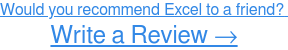Microsoft Excel can do just about any math problem for you instantly.

The problem many people struggle with though, is not knowing how to input these problems into Excel.

That’s where I come in.

I’ve spent a significant amount of time using Microsoft Excel. So, I can teach you how to subtract in Excelhow to add in Excel, and how to divide in Excel. Maybe a little bit about how to merge cells in Excel? The list goes on.

But in this particular article, I’m here to teach you how to multiply in Excel.

Now let’s get to it.# How to multiply in Excel

If you’re looking to multiply in Excel, you’ll need to know what Excel formulas are and how to write them.

Excel formulas are expressions used in Excel to display values that are calculated from the data you’ve entered and they’re generally used to perform various mathematical, statistical, and logical operations.

So before we jump into the specifics of how to multiply in Excel, here are a few reminders to keep in mind when writing Excel formulas:

• All Excel formulas should begin with the equal sign (=). This is a must in order for Excel to recognize it as a formula.
• The cells will display the result of the formula, not the actual formula. So by using cell references rather than just entering the data in the cell, if you need to go back later the values will be easier to understand.
• When using arithmetic operators in your formula, remember the order of operations (I personally reference the acronym PEMDAS: Parenthesis, Exponentiation, Multiplication, Division, Addition, Subtraction.) Below you’ll find an example of how the order of operations is applied to formulas in Excel.## How to multiply in a cell

To multiply numbers in a cell, you'll need to write your formula in the designated cell. Your formula should start with the equal sign (=), and contain the arithmetic operator (*) needed for the calculation. In this case, you want to multiply 10 by 5, so your formula is =10*5.Once you’ve entered the formula, press ‘Enter’ and your result will populate. In this example, the multiplication formula of =10*5 yields a result of 50 (see below).## How to multiply cells

For this, your data will need to be in its own cells so that you can use the cell references in the formula.

You can see below that a multiplication formula was written in cell C1 using cell references, but the data had to be entered separately in cells A1 and B1.Once you’ve entered the formula, press ‘Enter’ and your result will populate. In this example, the multiplication formula of =A1*B1 yields a result of 10 (see below).## How to multiply a range of cells

Both of the above values involved multiplying two variables. But did you know with Excel you can create formulas that will multiply a whole range of numbers for you? Pretty cool, huh?

To do this you can use a preset formula called a function – the PRODUCT function to be specific.

Once you’ve entered the data in separate cells, use the PRODUCT function (you can find this under ‘Insert Function’ or start typing =Product in the cell) and enter the range of the values you want to multiply.

Do this by entering the cell reference of the first cell in the range (A1), followed by a colon (:) and the cell reference of the last cell in the range (A5).After you have the function and cell range entered, press ‘Enter’ and your result will populate!

In this case, the values in cells A1 through A5 were multiplied, resulting in 120.TIP: Interested in more Excel tips? Learn how to divide in Excel

## You’re ready to multiply in Excel

In this article, we’ve covered everything you need to know about how to multiplying in Excel! Now grab your computer and dive into Excel to give it a try for yourself!

Did you enjoy this article? Check out how to lock cells in Excel and how to make a line graph in Excel!

Never miss a post.

Subscribe to keep your fingers on the tech pulse.